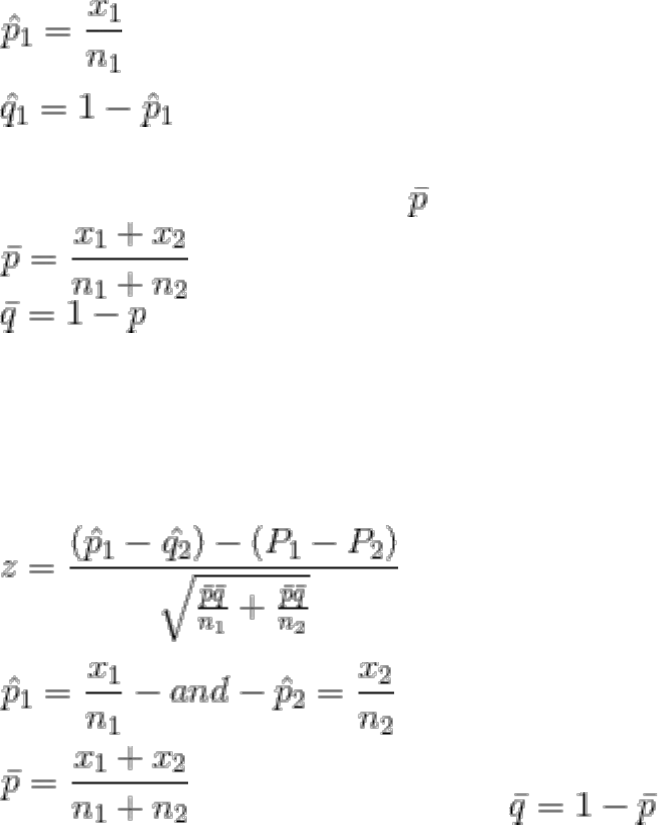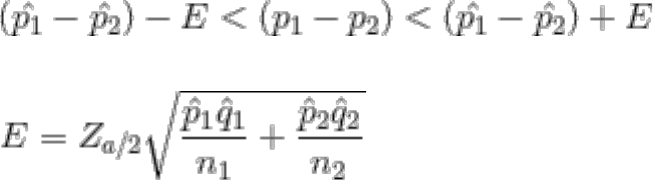Textbook Notes (270,000)
CA (160,000)
Brock U (1,000)
MATH (30)
Chapter

# MATH 1P98 Chapter Notes -Pennsylvania Route 309

Department
Mathematics and Statistics
Course Code
MATH 1P98
Professor
Lorna Deeth

This preview shows pages 1-2. to view the full 7 pages of the document.MATH 1P98-Chapter 9
Two Proportions
Objectives:
1. Test a claim about two population proportions or
2. Construct a confidence interval estimate of the difference between two population
proportions
Notation:
For Population 1 we let
P1= population proportion
N1= size of sample
X1= Number of Successes in the sample
(Sample proportion)
(complement of P(hat)1 )
Pooled Sample Proportion
The pooled sample proportion is given by and is given by
Requirements:
1. The sample proportions are from two simple random samples that are independent.
2. For each of the two samples there are atleast 5 successes and 5 failures
Test Statistic for Two Proportions
where p1 -p2 = 0 (null hypothesis)
Sample proportions
( pooled sample proportion) and
P-Value or Critical Value
Confidence Interval Estimate of p1-p2
The confidence interval estimate of the difference p1-p2 is

Only pages 1-2 are available for preview. Some parts have been intentionally blurred.Margin of error is given by:
Hypothesis Tests
-We only consider tests having a null hypothesis of P1=P2 ( so the null hypothesis is given as
H0:p1=p2.
-Can be done using a P-Value or Critical Value
-Find the p value of the test statistic, if its less than the test statistic then reject the null
hypothesis
Confidence Intervals
Use the format given earlier. If the confidence interval estimate does not include 0, we have
evidence suggesting that P1 and P2 have different values.
Cautions:
1. When testing a claim about two proportions, the critical value and p value method
are equivalent, but they are not equivalent to the confidence interval method. If you
want to test a claim about two population proportions use the p-value or critical
value method. If you want to estimate the difference between two population
proportions use a confidence interval.
2. Don’t test for equality of two population proportions by determining whether there
is an overlap between two individual confidence interval estimates of the two
individual population proportions. When compared to the confidence interval
estimate of p1-p2, the analysis of overlap between the two individual confidence
intervals is more conservative (by rejecting equality less often) and it has less
power(it is less likely to reject p1-p2 when in reality p1p2)
Two Means: Independent Samples
Part 1: Independent Samples with σ1 and σ2 unknown and Not assumed equal
This section involves two independent samples, and the following section deals with
samples that are deprendent. It is important to know the difference between independent
samples and dependent samples.
Independent: If the sample values from one population are not related to or somehow
naturally paired or matched with the sample values from the other population
Dependent: If the sample values are somehow matched, where the matching is based on
some inherent relationship. ( Each pair of sample values consists of two measurements from
the same subject such as before/after data or each pair of sample values consists of matched
pairs- such as husband/wife data where the matching is based on some meaningful
relationship.
Hypothesis Test/ Confidence Interval for two independent Means
Notation: